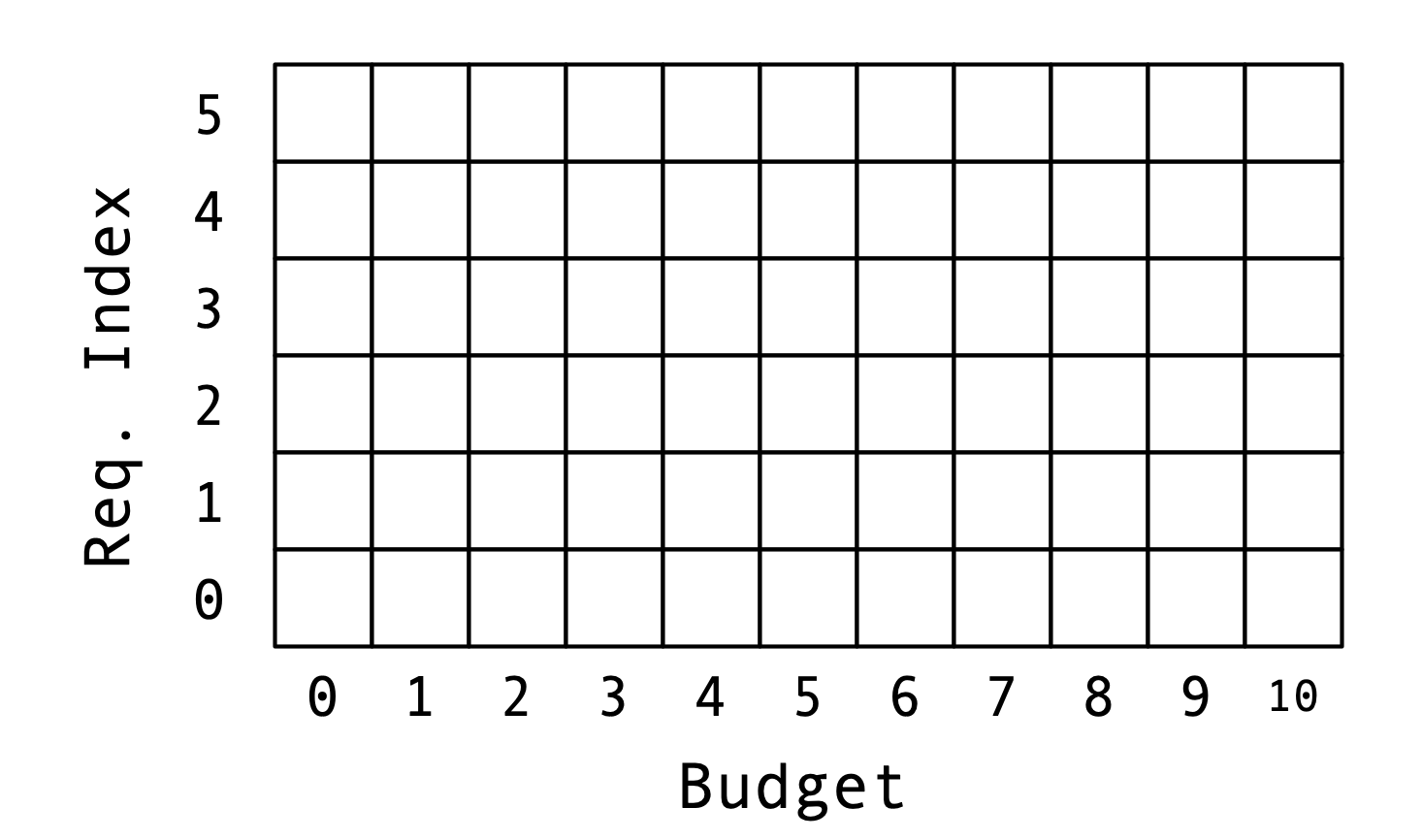Consider the instance of the weighted knapsack problem consisting of five requests with the following durations and values:

1. $$d_1 = 4$$, $$v_1 = 3$$
2. $$d_2 = 2$$, $$v_2 = 2$$
3. $$d_3 = 5$$, $$v_3 = 5$$
4. $$d_4 = 3$$, $$v_4 = 4$$
5. $$d_5 = 6$$, $$v_5 = 5$$

Suppose your total time budget is $$B = 10$$. Use the dynamic programming algorithm described in Lecture 24 to find the maximal value of a feasible collection of requests by hand. What is the collection that achieves the optimal value?

You may find the following grid helpful: